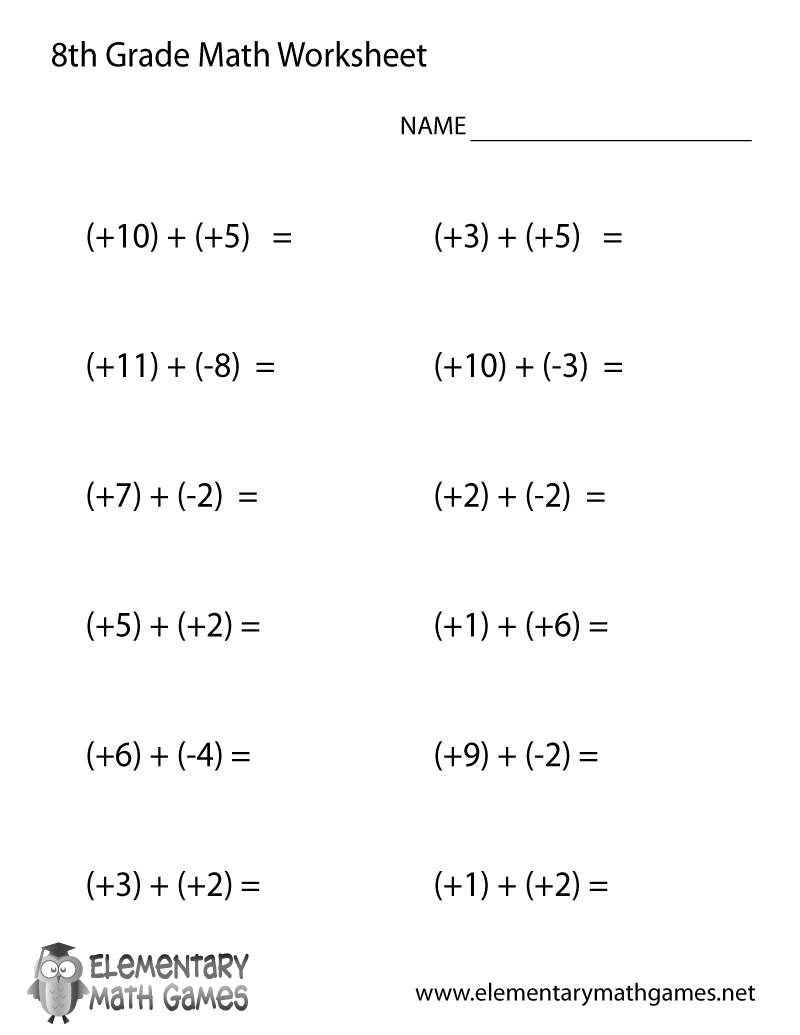## Learn and Practice Adding Positive and Negative Numbers

This eighth grade math worksheet is a great way for students to practice adding positive and negative numbers. Eighth graders have to solve 12 math addition problems.

Here are the two versions of this free eighth grade math worksheet: